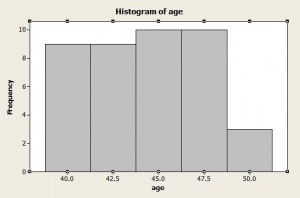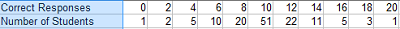# Sheppard’s Correction: DefinitionBinned data shown in a histogram.Sheppard’s Correction for Grouping Errors are adjustments to calculated sample moments for grouped data (i.e. data that has been binned). The correction is named after W.F. Shepard, who noted than when moments are calculated for continuous frequency distributions, it is assumed that the data is centered around the class interval midpoints. This erroneous assumption introduces “grouping errors” in calculation of moments. Grouping errors only happen in even moments (e.g. second and fourth moments), so the correction isn’t needed for odd-numbered moments.

## What Kind of Data Can Be Corrected?

Sheppard’s correction has some severe restrictions, which make it applicable to only a small range of distributions. The correction should only be made to data with the following characteristics:

• Frequencies should taper to zero in both the positive and negative direction. In other words, frequencies should be symmetrical and gradually taper off (like the behavior you would see in a normal distribution).
• Variables should be continuous. The method is not suited to discrete variables.
• Class intervals should be equal in width.
• Class intervals should be more than 1/20th of the total range .

In addition, Sharma’s Textbook of Sampling and Attributes note that the adjustment should not be made to:

## Sheppard’s Correction Formulas

• First moment (mean)—no correction needed.
• Second moment (variance): calculated estimate of the variance (m2 – (i2/12) where i is the class interval width.
• Third moment (skewness) — no correction needed.
• Fourth moment (kurtosis): calculated estimate for kurtosis (m4) – ½i2m2 + (7/240)i4

## ExampleThe distribution above has the following moments around the mean:

• m1 = 0
• m2 = 2.64
• m3 = 0.08
• m4 = 28.30

Use Sheppard’s correction for these moments. Note that the class interval for the distribution is 2.

Solution:

• m1 = 0; no correction needed.
• m2 = m2 – (i2/12) = 2.64 – 22/12 = .33 = 2.3.
• m3 = 0.08; no correction needed
• m4 = (m4) – ½i2m2 + (7/240)i4 = 28.30 – 5.28 + 0.12 = 23.14.

References:
A.K. Sharma. Textbook of Sampling and Attributes.

CITE THIS AS:
Stephanie Glen. "Sheppard’s Correction: Definition" From StatisticsHowTo.com: Elementary Statistics for the rest of us! https://www.statisticshowto.com/sheppards-correction/